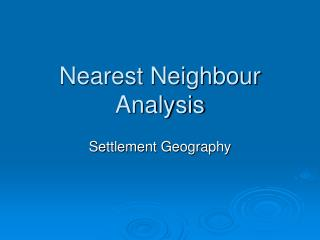DownloadDownload PresentationNearest Neighbour Analysis

# Nearest Neighbour Analysis

Download Presentation## Nearest Neighbour Analysis

- - - - - - - - - - - - - - - - - - - - - - - - - - - E N D - - - - - - - - - - - - - - - - - - - - - - - - - - -
##### Presentation Transcript

1. Nearest Neighbour Analysis Settlement Geography

2. Learning outcomes • To study the nearest neighbour analysis and how it determines patterns of settlements

3. Nearest Neighbour Analysis • Settlements often appear on maps as dots • The patterns of these dots are difficult to describe • Sometimes patterns are obvious: • Nucleated • Dispersed • However the pattern is likely to lie between these two extremes • Nearest neighbour helps to determine the pattern

4. The technique • Was devised by a botanist to determine plant distributions • Allows one region to be compared to another • It is only a technique and offers no explanation of patterns

5. Formula • The formula is • Rn= 2đ √n/A • Rn= The description of the distribution • đ = The mean distance between the nearest neighbours • n= the number of settlements in the study area • A= the area under study (km)

6. Rn • Rn is the description of the settlement distribution • This can be as follows: • Clustering- where dots are very close together. In extreme cases the value of Rn=0 • Random- distributions occur where there is no pattern at all. Rn= 1 • Regular- these are perfectly uniform. If ever found in reality they would have a value of 2.15

7. Nearest Neighbour values • See page 402 in Waugh